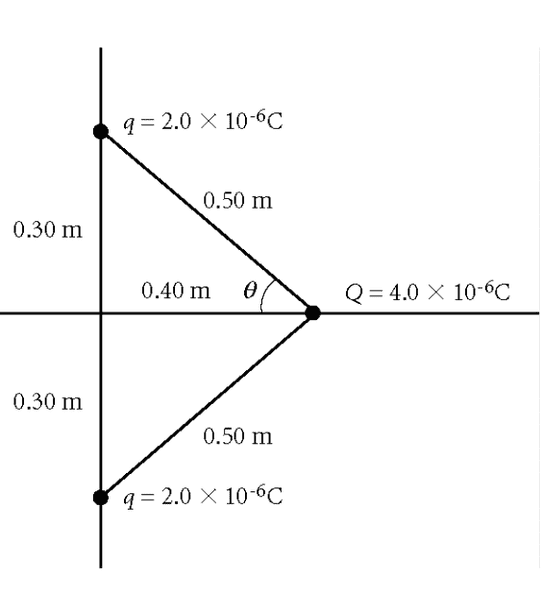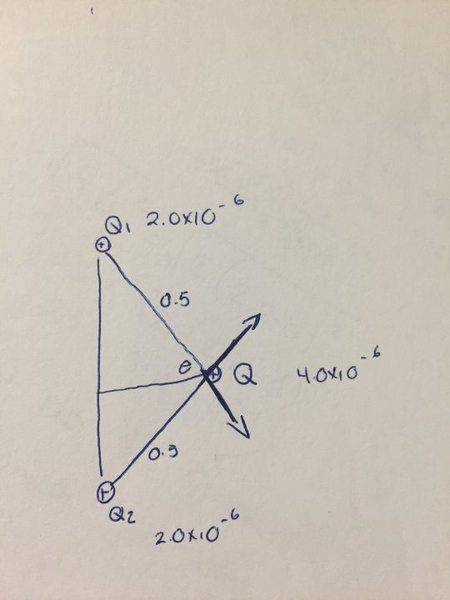# Electric Force and Superposition...Help

• Amaliahch
In summary, two equal positive charges, both q = 2.0 × 10−6 C, and a third charge, Q = 4.0 × 10−6 C, interact with each other. The magnitude of the electric force on Q can be found by calculating the X and Y components of each vector towards Q, taking into account the appropriate signs. The resulting values are 0.23N for the X component and 0.17N for the Y component.

## Homework Statement

Two equal positive charges, both q = 2.0 × 10−6 C, interact with a third charge, Q = 4.0 × 10−6 C, as shown in the figure above. What is the magnitude of the electric force on Q? (kC = 8.99 × 109 N•m2/C2)## Homework Equations

I'm confused about assigning signs when I get to the part of finding the X and Y components of each vector towards Q
I don't know if the values for the components I found are correctly signed... I believe are all positive

## The Attempt at a Solution

I find that the magnitude of both vectors since they are the same is O.29N
The X component of one vector is 0.23N and the Y component is 0.17N I think.

Amaliahch said:
I'm confused about assigning signs when I get to the part of finding the X and Y components of each vector towards Q
I don't know if the values for the components I found are correctly signed... I believe are all positive
It can help if you sketch in the force vectors on your diagram. Both of the 'q' charges will produce a force vector on the 'Q' charge. If you examine the vectors that you sketch then you should be able to tell from them the appropriate signs of their components.

I did but according to my sketch all four would be positive. I'm doing something wrong I'm not sure what it is because one of them is supposed to be negative, one of the y components

Can you post your diagram with the force vectors?

this is what i drewOkay. So looking at your two force vectors I see one pointing into quadrant 1 and the other into quadrant 4. You should be able to assign the signs of the vector components just by looking at the diagram. Take a close look at the y-components...

So it would be like this?
Q2 going to Q both components would be positive
Q1 going to Q the X component would be positive (cosine is positive in the 4th quadrant) and Y component would be negative( sine is negative in the 4th quadrant)

Amaliahch said:
So it would be like this?
Q2 going to Q both components would be positive
Q1 going to Q the X component would be positive (cosine is positive in the 4th quadrant) and Y component would be negative( sine is negative in the 4th quadrant)
Yup.

Thank you!